Next: Convolution in the frequency Up: SYMMETRIES Previous: SYMMETRIES

## Plot interpretation

Now we will get away from the ends and think about what is in the middle of signals. Figure 7 shows even functions in both time and frequency domains. This figure was computed with the matrix equations (3) and (4). Displaying both the left and right halves of each function wastes half the paper; equivalently, for a fixed amount of paper, it wastes half the resolution. Typically, only the left half of each function is displayed. Accepting this form of display, we receive a bonus: each figure can be interpreted in two more ways.

Since imaginary parts are not shown, they are arbitrary. If you see only half of an axis, you cannot tell whether the function is even or odd or neither. A frequently occurring function is the causal'' function, i.e., the function that vanishes for t<0. Its even part cancels its odd part on t<0. The ro transforms to an IO, which, being imaginary, is not shown.

The third interpretation of these displays is that the frequency function is one-sided, and the time signal is complex. Such signals are called analytic signals.'' For analytic signals, RE extinguishes RO at negative,and the imaginary even part, ie, is not displayed.

 In summary, plots that show only half the axes can be correctly interpreted in three ways:  left side right side eveneven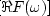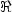causal(t)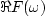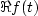OneSidedHow can we compute these invisible imaginary parts? Their computation is called Hilbert transform.'' Briefly, the Hilbert transform takes a cosinusoidal signal (like the real part of the FT of a delayed impulse, i.e.,) and converts it to a sinusoidal signal of the same amplitude (like the imaginary part of a delayed impulse,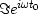).Next: Convolution in the frequency Up: SYMMETRIES Previous: SYMMETRIES
Stanford Exploration Project
10/21/1998